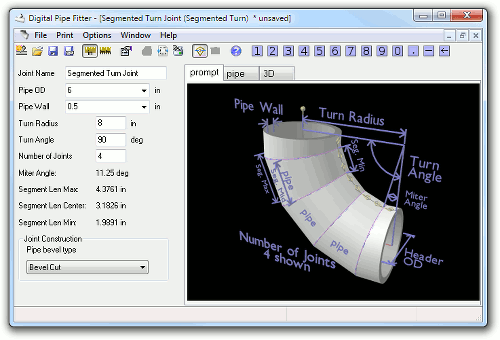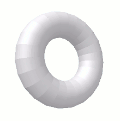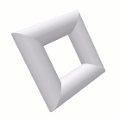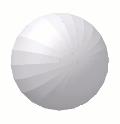# Segmented Turn Jointback to other joints

A Segmented Turn Joint is a way to create an elbow or smooth curve using multiple segments of straight pipe.

A single template is generated that can be used to cut each segment. The first and last segments can be any length so only use one cutline for these segments.

## Parameter Constraints:

Joint Name:  name of this joint which is unique to this project or currently opened joints

Pipe OD: (Outside Diameter) must be a positive number

Pipe Wall: (Wall Thickness) must be a positive number, less than half Pipe OD

Turn Angle: more than 0 deg. and less than 180 deg.

Number of Joints: The number of joints used to create the curve. A positive number.

Additional output fields are shown under the input parameters for the user's convenience.

Miter Angle: is the angle to cut the ends of the pipe to create this joint.

Segment Len Max: is the length of the segment at its longest point, which corresponds to the distance between joints measured on the outside of the curve.

Segment Len Center: is the length of the segment at the midpoint, which corresponds to the distance between joints measured on the side of the curve.

Segment Len Min: is the length of the segment at its shortest point, which corresponds to the distance between joints measured on the inside of the curve.

## Creative Uses:

 Setting Number of Joints to 1 makes this joint digress into something similar to a Miter Joint. Number of Joints = 1 and Turn Angle = 60It can even make a doughnut. Turn Angle = 360Regular Polygons Turn Angle = 360 and Number of Joints=4Basket Ball? Turn Radius = 0 and Turn Angle=360